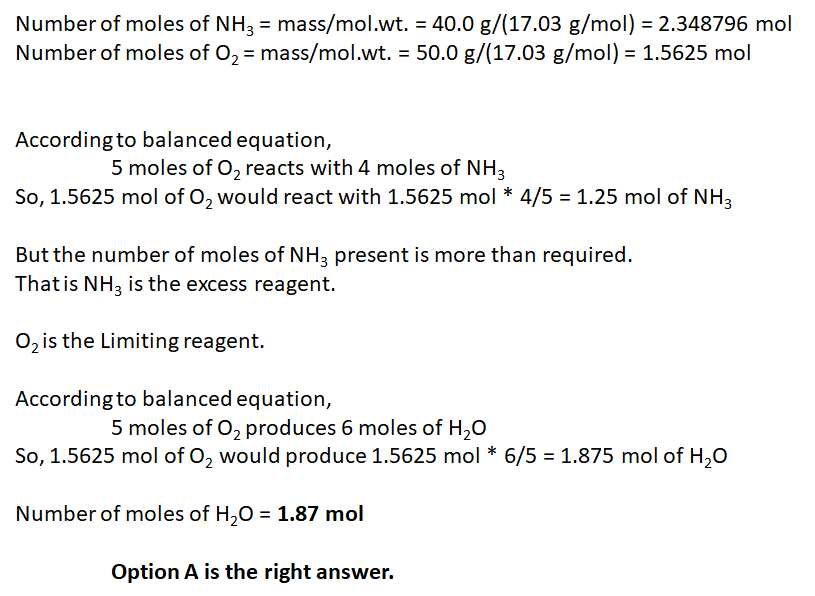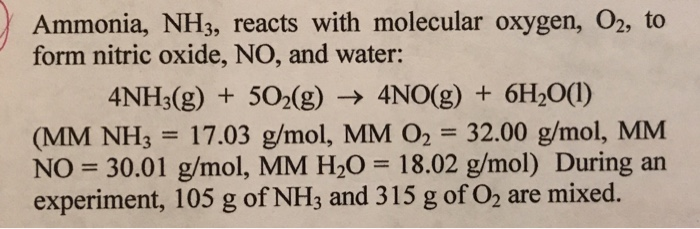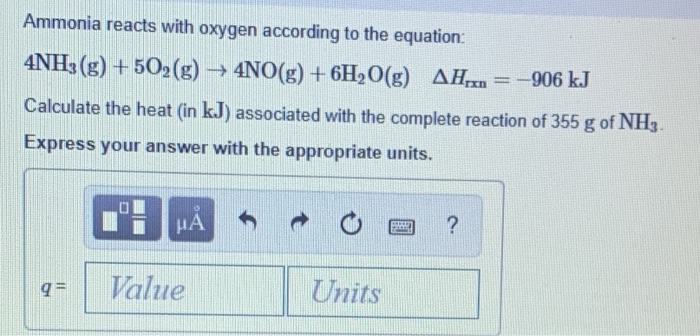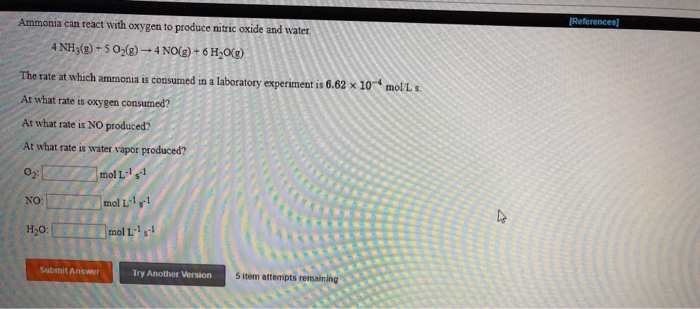Question

# Ammonia reacts with oxygen to form nitric oxide and water vapor: 4NH3 +5O2 → 4NO + 6H2O

Ammonia reacts with oxygen to form nitric oxide and water vapor:

4NH3 +5O2 → 4NO + 6H2

What is the theoretical yield of water, in moles, when 40.0g NH3 and 50.0g O2 are mixed and allowed to react:

•  1.87 mol

•  3.53 mol

•  1.57 mol

•  1.30 mol

•  None of these

Number of moles of NH3= mass/mol. wt .=40.0 g/ (17.03 g/mol)=2.348796 mol

Number of moles of O2= mass / mol . wt .=50.0 g /(17.03 g / mol)=1.5625 mol

According to balanced equation,

5 moles of O2 reacts with 4 moles of NH3

So, 1.5625 mol of O2 would react with 1.5625 mol * 4 / 5 = 1.25 mol of NH3

But the number of moles of NH3 present is more than required.

That is NH3 is the excess reagent.

O2 is the Limiting reagent.

According to the balanced equation,

5 moles of O2 produces 6 moles of H2O

So, 1.5625 mol of O2 would produce 1.5625 mol * 6 / 5=1.875 mol of H2O

The number of moles of H2O = 1.87 mol

Option A is the correct answer.To calculate the theoretical yield of water (H2O) in the given reaction, we first need to identify the limiting reactant. The limiting reactant is the reactant that is completely consumed in the reaction, thereby limiting the amount of products that can be formed.

Let's first calculate the number of moles of NH3 and O2 present in the given reaction:

Number of moles of NH3 = 40.0 g / (17.03 g/mol) = 2.35 mol

Number of moles of O2 = 50.0 g / (32.00 g/mol) = 1.56 mol

According to the balanced chemical equation, 4 moles of NH3 react with 5 moles of O2 to produce 6 moles of H2O. Therefore, if NH3 is the limiting reactant, the theoretical yield of water can be calculated as:

Theoretical yield of H2O = (2.35 mol NH3) x (6 mol H2O / 4 mol NH3) = 3.53 mol H2O

Similarly, if O2 is the limiting reactant, the theoretical yield of water can be calculated as:

Theoretical yield of H2O = (1.56 mol O2) x (6 mol H2O / 5 mol O2) = 1.87 mol H2O

Since the calculated theoretical yield of H2O using NH3 as the limiting reactant is higher than that using O2 as the limiting reactant, NH3 is the limiting reactant in this case. Therefore, the theoretical yield of water is 3.53 mol.

Hence, the correct answer is 3.53 mol.

1. To find the theoretical yield of water (H2O) in moles, we need to follow the stoichiometry of the balanced equation:

2. 4NH3 + 5O2 → 4NO + 6H2O

3. First, let's calculate the number of moles for NH3 and O2 using their respective masses and molar masses:

4. Molar mass of NH3 (Ammonia) = 14.01 g/mol + 3(1.01 g/mol) = 17.03 g/mol

5. Moles of NH3 = mass of NH3 / molar mass of NH3 = 40.0 g / 17.03 g/mol ≈ 2.35 mol

6. Molar mass of O2 (Oxygen) = 2(16.00 g/mol) = 32.00 g/mol

7. Moles of O2 = mass of O2 / molar mass of O2 = 50.0 g / 32.00 g/mol ≈ 1.56 mol

8. Now, we can determine the limiting reactant by comparing the moles of NH3 and O2. The reactant that produces the least amount of product is the limiting reactant.

9. From the balanced equation, we can see that the stoichiometric ratio between NH3 and H2O is 4:6 (4 moles of NH3 produce 6 moles of H2O).

10. Moles of H2O formed from NH3 = 2.35 mol NH3 × (6 mol H2O / 4 mol NH3) ≈ 3.52 mol H2O

11. From the balanced equation, the stoichiometric ratio between O2 and H2O is 5:6 (5 moles of O2 produce 6 moles of H2O).

12. Moles of H2O formed from O2 = 1.56 mol O2 × (6 mol H2O / 5 mol O2) ≈ 1.87 mol H2O

13. Since the theoretical yield is limited by the reactant that produces the least amount of product, the limiting reactant is O2, and the theoretical yield of water (H2O) is approximately 1.87 moles.

source: me.

#### Earn Coins

Coins can be redeemed for fabulous gifts.

Similar Homework Help Questions
• ### Ammonia reacts with diatomic oxygen to form nitric oxide and water vapor:

Always show your work. No credit will be given for any answers (even if they are correct) for which work is not shown. Ammonia reacts with diatomic oxygen to form nitric oxide and water vapor: 4NH3 + 502 ---> 4NO + 6H2O What is the theoretical yield of water, in moles, when 40.0 g NH3 and 50.0g, O2 are mixed and allowed to react?

• ### Ammonia reacts with oxygen to form nitric oxide and water vapor: 4NH3 + 5O2 ---> 4NO...

Ammonia reacts with oxygen to form nitric oxide and water vapor: 4NH3 + 5O2 ---> 4NO + 6H2O When 20.0 g NH3 and 50.0 g O2 are allowed to react, which is the limiting reagent? A) NO B) O2 C) H2O D) no reagent is limiting E) NH3 What is the coefficient for O2 when the following combustion reaction of a hydrocarbon is balanced? ___C7H14 + ___ O2 ---> ___ CO2 + __ H2O A) none of these B) 10...

• ### 1) Ammonia, NH3, reacts with molecular oxygen, O2, to formnitric oxide, NO, and water:4NH3(g)...1) Ammonia, NH3, reacts with molecular oxygen, O2, to form nitric oxide, NO, and water:4NH3(g) + 5O2(g) = 4NO (g) +6H2O(l)A. What is the limiting reactant and what is the theoretical yield of NO?B. What is the theoretical yield of H2O?C. How many grams of excess reagent will be left over?D. If the actual yield of NO had been 91 g, what would be the percent yield of the reaction

• ### 1. Ammonia reacts with oxygen according to the equation 4NH3(g)+5O2(g)→4NO(g)+6H2O(g),ΔHrxn=−906 kJ Calculate the heat...

1. Ammonia reacts with oxygen according to the equation 4NH3(g)+5O2(g)→4NO(g)+6H2O(g),ΔHrxn=−906 kJ Calculate the heat (in kJ) associated with the complete reaction of 155 g of NH3. 2. What mass of butane in grams is necessary to produce 1.5×103 kJ of heat? What mass of CO2 is produced? Assume the reaction to be as follows: C4H10(g)+132O2(g)→4CO2(g)+5H2O(g),ΔHrxn=−2658 kJ

• ### Part A Ammonia reacts with oxygen according to the equation 4NH3(g)+5O2(g)→4NO(g)+6H2O(g),ΔHrxn=−906 kJ Calculate the h...

Part A Ammonia reacts with oxygen according to the equation 4NH3(g)+5O2(g)→4NO(g)+6H2O(g),ΔHrxn=−906 kJ Calculate the heat (in kJ) associated with the complete reaction of 155 g of NH3. Part B What mass of butane in grams is necessary to produce 1.5×103 kJ of heat? What mass of CO2 is produced? Assume the reaction to be as follows: C4H10(g)+132O2(g)→4CO2(g)+5H2O(g),ΔHrxn=−2658 kJ

• ### The first step in the Oswald process for producing nitric acid is 4NH+5o2- 4NO + 6H2O...

The first step in the Oswald process for producing nitric acid is 4NH+5o2- 4NO + 6H2O if 132g of ammonia reacts with 132g of oxygen A) how many grams of nk itric oxide(No) will be produced B) which compound is limiting reagent C) what is the % yield of this reaction if actual yield of NO is 67.Og?

• ### Ammonia reacts with oxygen according to the equation: 4NH3(g) +502(g) → 4NO(g) + 6H2O(g) AH.,.. =...Ammonia reacts with oxygen according to the equation: 4NH3(g) +502(g) → 4NO(g) + 6H2O(g) AH.,.. = 4906 kJ Calculate the heat (in kJ) associated with the complete reaction of 355 g of NH3. Express your answer with the appropriate units. Value Units

• ### (References] Ammonia can react with oxygen to produce nitric oxide and water 4 NH3(g) +502() - 4 NO(g) + 6H2O() mol...(References] Ammonia can react with oxygen to produce nitric oxide and water 4 NH3(g) +502() - 4 NO(g) + 6H2O() mol L s. The rate at which ammonia is consumed in a laboratory experiment is 6.62 x 10 At what rate is oxygen consumed? At what rate is NO produced At what rate is water vapor produced? moll's1 NO: mol Lly HO: moll Submit Answer Try Another Version 5 item attempts remaining

• ### As a first step in the production of nitric acid, ammonia is oxidized to form nitric...

As a first step in the production of nitric acid, ammonia is oxidized to form nitric oxide: 4NH3 + 5O2→4NO + 6H2O A side reaction also occurs: 2NH3 +3/2O2→N2 +3H2O A reactor is designed to carry out this reaction isothermally at 600°C, with pure oxygen fed to the reactor in 50% excess. At these conditions, all components are in the gas phase. The conversion of ammonia is 80%, and the selectivity ratio of NO to N2 in the product stream...

• ### Ammonia (NH3) chemically reacts with oxygen gas (O2) to produce nitric oxide (NO) and water (H2O)...

Ammonia (NH3) chemically reacts with oxygen gas (O2) to produce nitric oxide (NO) and water (H2O) . What mass of oxygen gas is consumed by the reaction of 8.8g of ammonia? Be sure your answer has the correct number of significant digits